## Engineering Notes by Oriental Motor

Other than torque or speed, another factor should be considered when sizing motors.Have you ever wondered why the wheels and tires of a racing bicycle are different from a mountain bike?  That is because racing bicycles use lighter, thinner wheels for performance.  The lighter, thinner wheels reduce the moment of inertia of the wheels; making it a little easier to peddle.  In racing where milliseconds count, a little means a lot.

 What is "Inertia"?

Inertia comes from the Latin word, iners, meaning idle or sluggish.  Inertia is defined as the resistance of any physical object to any change in its speed.  The bigger the inertia, the more resistant it will be to acceleration or deceleration.

Load inertia, or moment of inertia, is the resistance of any physical object to any change in its speed from the perspective of the rotational axis.  For a rotary load, it's the product of its mass and the square of the perpendicular distance of the mass from the axis.  Load inertia is typically referred to as "J".

 What is "Permissible Load Inertia" and "Inertia Ratio"?

Motors cannot drive an infinite amount of load inertia.  Manufacturers often offer permissible load inertia or an inertia ratio value for motors to help with motor sizing.  Permissible load inertia values are guidelines typically given for AC and brushless motors (examples are given at the end of the post).  Inertia ratios are typically given for stepper or servo motors, and they are calculated by dividing the total amount of load inertia (or reflected load inertia if geared) by the rotor inertia of the motor.  If these values are exceeded, the motor may miss steps, stall, or vibrate.  Closed-loop motors can handle a larger inertia ratio than open-loop motors.

Example: Recommended Permissible Inertia Ratios

 Motor Type Frame Size (mm) Frame Size (NEMA) Inertia Ratio Open-Loop Stepper Motors 20, 28, 35 8, 11, 14 5:1 or less Open-Loop Stepper Motors 42, 50, 56.4, 60, 85 17, 20, 23, 24, 34 10:1 or less Closed-Loop Stepper Motors - - 30:1 or less Servo Motors (Auto Tuning) - - 50:1 or less Servo Motors (Manual Tuning) - - 100:1 or less

 TIP: If you need to exceed the load inertia ratio... Remember that this is a safe guideline and these values can be exceeded with the right setup.  I remember talking to someone who was building slot machines for casinos (the old kind with a lever).  His team was using a stepper motor to spin the cylinders that determined if you kept your day job or not.  With the right motion profile, a slow acceleration and deceleration ramp, and repeated testing, they were able to use a motor that exceeded these permissible values...by a lot.

Let's take a look at some common examples to see how the load inertia is calculated.  First, the fundamental equation for inertia (J) is shown below.

Fundamental Inertia (J) Equation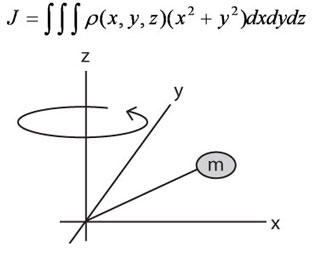Don't worry.  There are simplified forms of this formula.  Five different simplified equations for five common loads (objects) are shown below for solid cylinder, hollow cylinder, rectangular object, rectangular object with off-center axis, and object in linear motion.

Choose the appropriate equation based on:

• Shape of load (object) in motion
• Rotational axis (x or y)
• Details provided (do you have the weight of the load?)

For example, if weight is provided, and you're calculating for a solid cylinder rotating about its x axis, use the first equation (Jx) below (with mass "m").  If the weight is not provided, but you have the diameter, thickness, and material density of the load, then the load inertia can be calculated with the second equation (Jx) below (with density "p").

Inertia of a Cylinder or Disc (as from the x or y axis)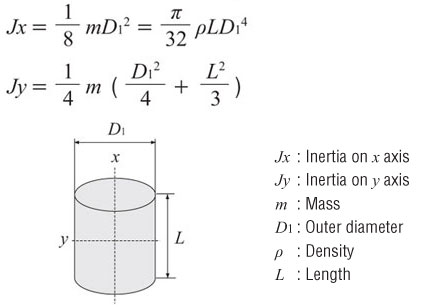Inertia of a Hollow Cylinder (as from the x or y axis)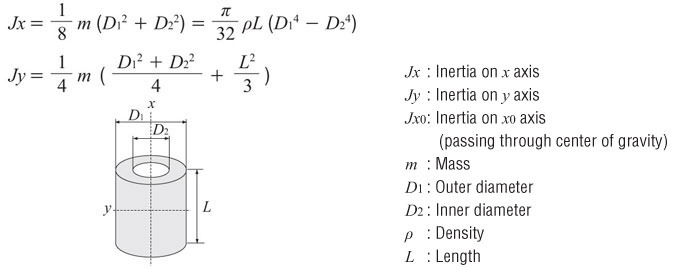Inertia of a Rectangular Object (as from the x or y axis)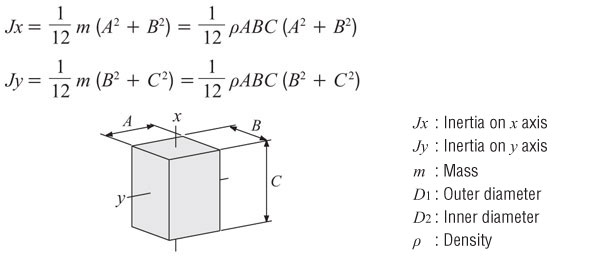Inertia of a Rectangular Object with Off-Center AxisInertia of an Object in Linear MotionUnit of Measure

The units of inertia are commonly used in two ways, oz-in² and oz-in-sec². The former includes gravity, the latter only mass.  Theoretically, inertia is a factor of mass so it should not include gravity, however, practically we can not easily measure mass on the earth.

Oriental Motor commonly provides inertia in oz-in². Then, when we calculate the acceleration torque, we divide the total inertia by gravity.

Gravity = 386 in/sec²

• oz-in² = Inertia based on weight
• oz-in-sec² = inertia based on mass

Conversion from oz-in² to oz-in-sec²To complete these calculations, you may need some extra info such as material density to complete your calculations.  This is necessary to calculate the weight of an object.  More can be found with a simple online search.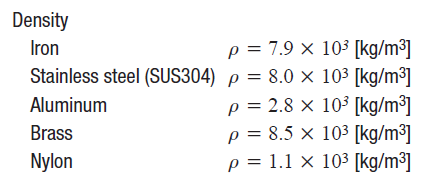Try to calculate the load inertia for the following application.  Which parts do you need to calculate for?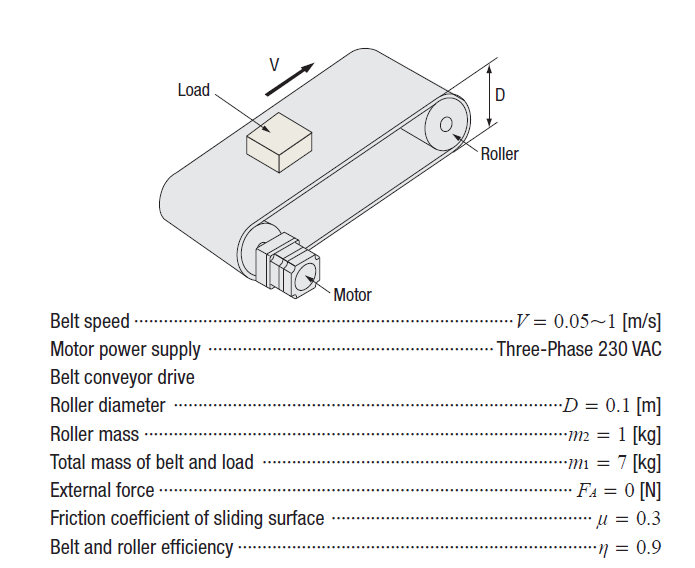You'll need to add up all the load inertia values of all components driven by the motor.  This includes the load, the belt, and the rollers.  You'll need to use 2 different equations.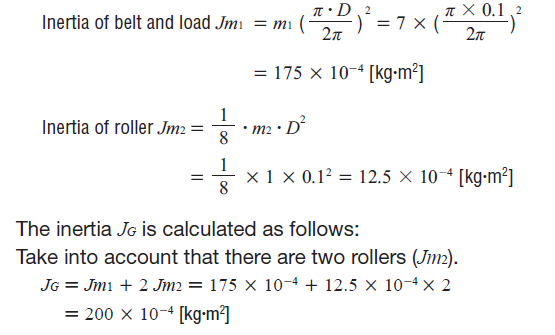From what we've learned in, here's the load torque calculation.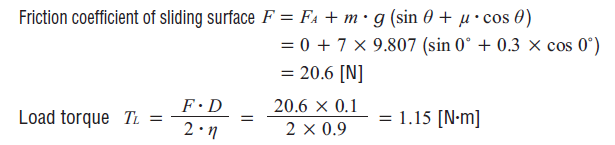Dealing with Large Load Inertia?  Use a Geared Motor

If you are dealing with a large amount of load inertia, there is a simple way to reduce it exponentially.  The load inertia is reduced by the gear ratio squared.  The resulting value is the reflected load inertia, which is the load inertia at the motor shaft (as opposed to load inertia at the gearhead shaft).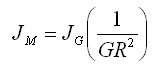If you're interested in learning more, here's a white paper that discusses how to use gearheads to reduce load inertia.  This is specifically for stepper motors.Where Can I Find "Permissible Load Inertia"?

Now that you have calculated your total load inertia value, how do you find a motor that can handle it?

Here's an example of a permissible load inertia chart for a BLE2 Series 200/400 W brushless motor (from our catalog).  Since we've already calculated the maximum permissible load inertia values for each gear ratio, you don't have to.  Try not to exceed these values.  The motors may still work if these values are exceeded, but it cannot be guaranteed.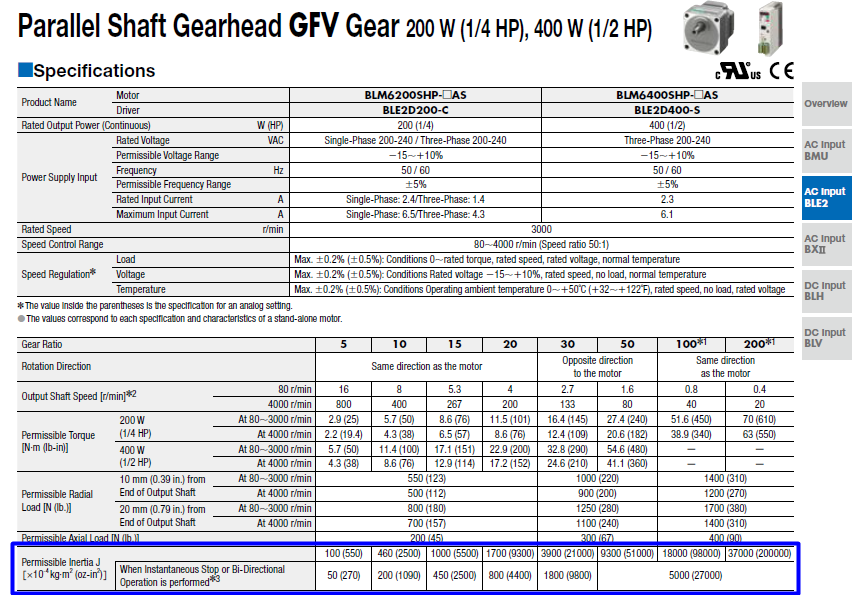If you don't have our catalog, we also list the permissible load inertia value on the website.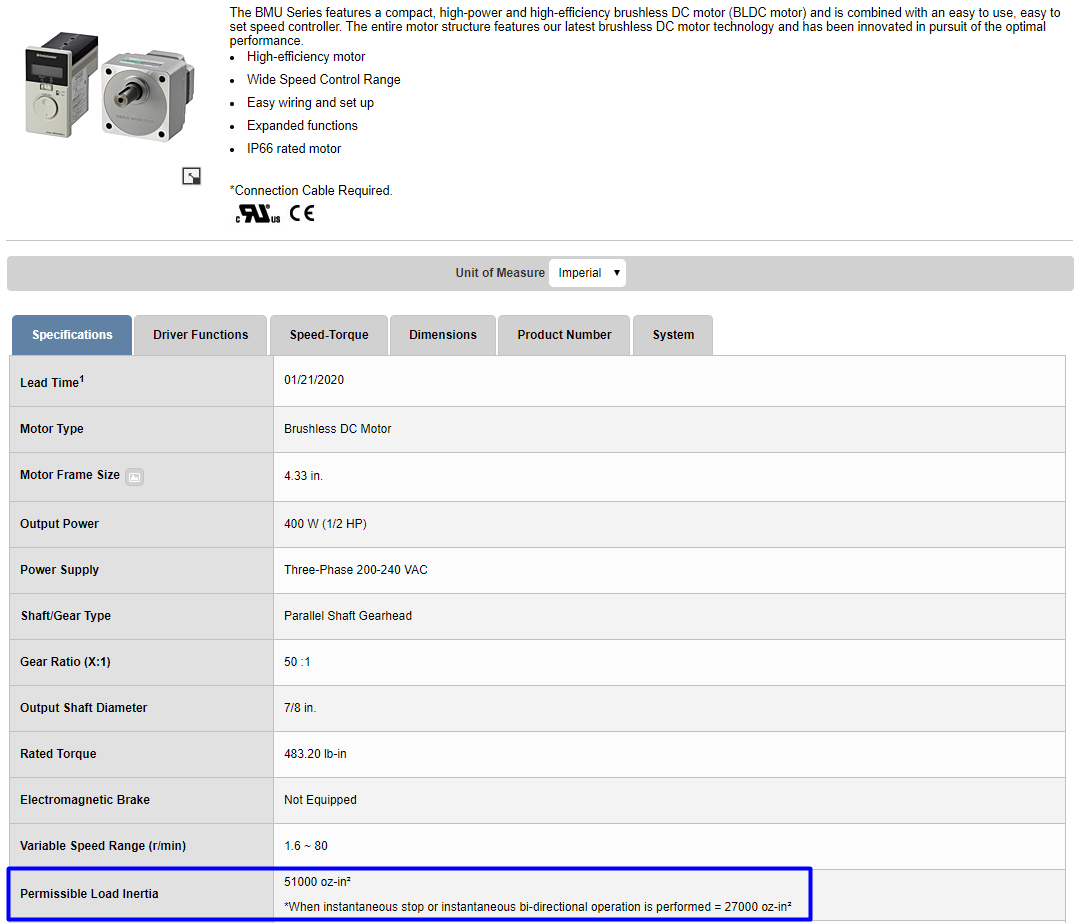For stepper motors or servo motors, permissible load inertia values are not published, so please use recommended inertia ratio guidelines.

That's it for load inertia for now.  Remember that load inertia is just one of three calculations necessary for a successful motor sizing (don't forget torque and speed).  In the next Motor Sizing Basics post, I will explain how load inertia affects another component of torque - acceleration torque, which is also important in determining the total torque requirement for an application (and is the main reason to why racing bikes peddle easier than regular bikes).

Need a refresher?  Here's the motor sizing white paper (from the last post).In the next post, I will explain how to calculate acceleration torque, RMS torque, and speed.Here's the last post:TIP: Is there an easier way to size motors? The thing to remember about motor sizing is that the result is only as good as the data.  Make sure the values used for the calculation are as accurate as possible.  The more guessing you do, the larger the safety factor you'll need to use at the end.  Just like in the real world, there will be some unknowns. Example: Belt Conveyor#### Written by Johann Tang

Johann Tang is a Product Specialist at Oriental Motor USA Corp. with over 15 years of knowledge and experience supporting applications of various types of electric motors, gearheads, actuators, drivers, and controllers.

### Recent Posts